Courses

# Pie Graph - Practice Test (With Solutions) CAT, Common Admission Test

## 12 Questions MCQ Test | Pie Graph - Practice Test (With Solutions) CAT, Common Admission Test

Description
This mock test of Pie Graph - Practice Test (With Solutions) CAT, Common Admission Test for LR helps you for every LR entrance exam. This contains 12 Multiple Choice Questions for LR Pie Graph - Practice Test (With Solutions) CAT, Common Admission Test (mcq) to study with solutions a complete question bank. The solved questions answers in this Pie Graph - Practice Test (With Solutions) CAT, Common Admission Test quiz give you a good mix of easy questions and tough questions. LR students definitely take this Pie Graph - Practice Test (With Solutions) CAT, Common Admission Test exercise for a better result in the exam. You can find other Pie Graph - Practice Test (With Solutions) CAT, Common Admission Test extra questions, long questions & short questions for LR on EduRev as well by searching above.
QUESTION: 1

### Refer to the following pie chart and solve the questions based on it.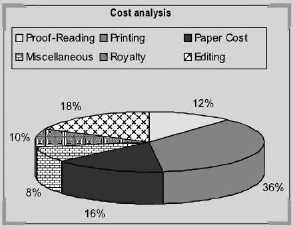What is the central angle showing the cost of paper?

Solution:

Required Angle = (16/100 x 360) = 57.6°

QUESTION: 2

### Refer to the following pie chart and solve the questions based on it.The royalty on the book is less than the editing expenditure by

Solution:

If the editing charges are Rs 18, royalty is Rs 10. On Rs 18, it is less by 8. On Rs 100, it is less by (8/18 x 100)% = 44.44%

QUESTION: 3

### Refer to the following pie charts and solve the questions based on it* The pie charts given below represent the market share of different players in the electronics market in the year 1995 and 2000 A new player F has entered the market in the given period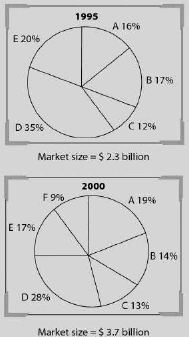What is the percentage increase in the sales of A in the year 2000 over 1995?

Solution:

There are two successive increases—First is the total increase of 60% approx.( \$ 3.7 bn in 2000 from \$ 2.3 billion in 1995) and second is the percentage share of A by 20% approx. Hence total percentage increase = 92%.

QUESTION: 4

Refer to the following pie charts and solve the questions based on it* The pie charts given below represent the market share of different players in the electronics market in the year 1995 and 2000 A new player F has entered the market in the given periodIf the new entrant F has grown at the expense of B, C, and E, what would be the change in the net value of sales of E in the year 2000, had F not entered the market?

Solution:

Since we do not know what part of market share of B. C and E has been eaten up by F, so cannot be determined.

QUESTION: 5

The following pie-chart shows the percentage distribution of the expenditure incurred in publishing a book. Study the pie-chart and the answer the questions based on it.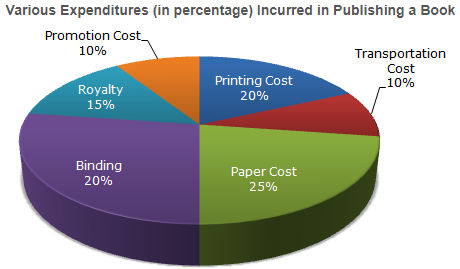Various Expenditures (in percentage) Incurred in Publishing a BookIf for a certain quantity of books, the publisher has to pay Rs. 30,600 as printing cost, then what will be amount of royalty to be paid for these books?

Solution:

Let the amount of Royalty to be paid for these books be Rs. r.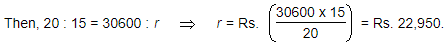QUESTION: 6

The following pie-chart shows the percentage distribution of the expenditure incurred in publishing a book. Study the pie-chart and the answer the questions based on it. What is the central angle of the sector corresponding to the expenditure incurred on Royalty?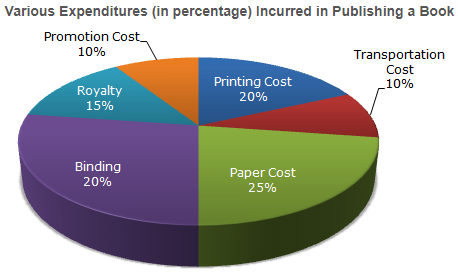Solution: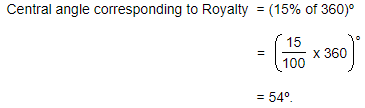QUESTION: 7

Refer to the following pie charts and solve the questions based on It.
The two pie charts given below provide the expenses of two families: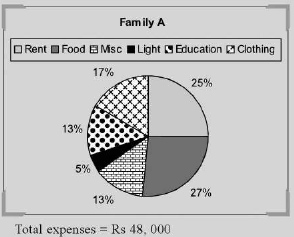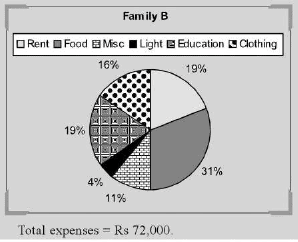Both the families decide to double the total expenditure keeping the pattern of spending the same as given above. What will be the new ratio of expenditure on food between family A and family B?

Solution:

If the percentage increase in the expenditure of both the families is the same then the ratio will be the same

QUESTION: 8

Refer to the following pie charts and solve the questions based on It.
The two pie charts given below provide the expenses of two families:What will be the expenses on light by family A, as a percentage of expense on light by family B?

Solution:

By visual inspection we can see that option (c) is the correct answer.

QUESTION: 9

Refer to the following pie charts and solve the questions based on It.
The two pie charts given below provide the expenses of two families:Under how many heads are the expenses of family B more than the expenses of family A?

Solution:

By visual inspection we can see that the correct answer is option (b).

QUESTION: 10

Refer to the following pie charts and solve the questions based on it.
The following pie charts represent the budget expenditure of certain countries on various sectors for the year in 2007: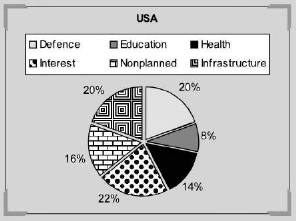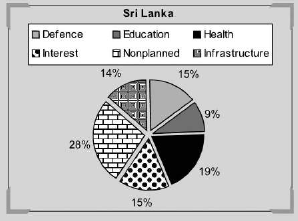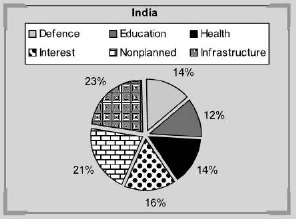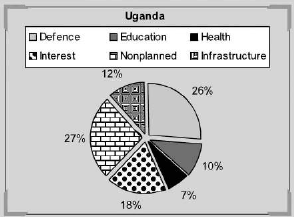A country is said to be progressive if its education, health and infrastructure expenditures are in the top four expenditure sectors. How many of the four countries are progressive?

Solution:

By visual inspection we can see that option (a) is the correct answer.

QUESTION: 11

Refer to the following pie charts and solve the questions based on it.
The following pie charts represent the budget expenditure of certain countries on various sectors for the year in 2007:If a country is under military rule, it will spend maximum on defence and minimum on either health or education. How many of the above countries are under military rule?

Solution:

Understand the logic that “If a country is under military rule, it will spend maximum on defence and minimum on either health or education” does not mean that if a country is spending maximum on defence and minimum on either health or education, then it will under military rule.

QUESTION: 12

Refer to the following pie charts and solve the questions based on it.
The following pie charts represent the budget expenditure of certain countries on various sectors for the year in 2007:If the budgetary expenditures of all the four countries are combined, which sector will account for the maximum expenditure?

Solution:

Since individual expenditures of the countries are not given, we cannot determine it.